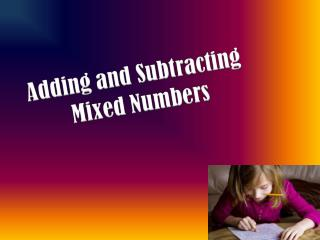DownloadDownload PresentationFind the common denominator. Make equivalent fractions.

# Find the common denominator. Make equivalent fractions.

Télécharger la présentation## Find the common denominator. Make equivalent fractions.

- - - - - - - - - - - - - - - - - - - - - - - - - - - E N D - - - - - - - - - - - - - - - - - - - - - - - - - - -
##### Presentation Transcript

1. Find the common denominator. Make equivalent fractions.

2. Find the common denominator. Make equivalent fractions.

3. Find the common denominator. Make equivalent fractions. 1 10 10 10 6

4. Find the common denominator. Make equivalent fractions. 3 15 15 15 5

5. Find the common denominator. Make equivalent fractions. 5 8 8 8 8

6. Find the common denominator. Make equivalent fractions.

7. Find the common denominator. Make equivalent fractions.

8. Find the common denominator. Make equivalent fractions.

9. Find the common denominator. Make equivalent fractions.

10. S O L V E